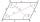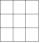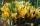RWY

Calculate the opposite direction of the runway 13.

Runways are named by a number between 01 and 36, which is generally one tenth of the azimuth of the runway's heading in degrees: a runway numbered 09 points east (90°), runway 18 is south (180°), runway 27 points west (270°) and runway 36 to the north (360° rather than 0°).

A runway can normally be used in both directions, and is named for each direction separately.

Result

x =  31

Solution:Leave us a comment of this math problem and its solution (i.e. if it is still somewhat unclear...):Be the first to comment!Next similar math problems:

1. ParallelogramIn the parallelogram we know one internal angle 67°33`. Calculate the other internal angles.
2. Addition of Roman numbersAdded together and write as decimal number: LXVII + MLXIV
3. Roman numerals +Add up the number writtens in Roman numerals. Write the results as a decimal number.
4. Roman numerals 2+Add up the number writtens in Roman numerals. Write the results as a roman numbers.
5. Table 3x3Table with numbers 3x3 in the first row 66,24,33 in the second row 57,? 19 in the third row 18,45,60 What number comes ar mark ?. Maybe the numbers: 22,46,45,47
6. Adding mixed numbersAdd this two mixed numbers: 1 5/6 + 2 2/11=
7. Addition of mixed numeralsAdd two mixed fractions: 2 4/6 + 1 3/6
8. Adding mixed numerals3 3/4 + 2 3/5 + 5 1/2 Show your solution.
9. Angles 1It is true neighboring angles have not common arm?
10. Combine / add termCombine like terms 4c+c-7c
11. Obtuse angleWhich obtuse angle is creating clocks at 17:00?
12. Evaluate expression 2Evaluate expression with negatives: (-3)+4+(-8)+(-6)+4+(-1)
13. CrocusesThe garden grow daffodils, crocuses and roses. 1400 daffodils, crocuses is 462 more and roses is 156 more than crocuses. How many are all the flowers in the garden?At a weather centre, the temperature at midnight was -2 degree Celsius and by noon it had raised 4 degree Celsius. What is the new temperature?This morning it was 80 degrees F outside the temperature increased 12 degrees F. What is the temperature now?At the florist are 50 tulips and 5 times less roses. How many flowers are in flower shop?In the morning temperature was 110°F. By noontime it has gone up by 15°F. What was the noon temperature. (Give your answer in °F)